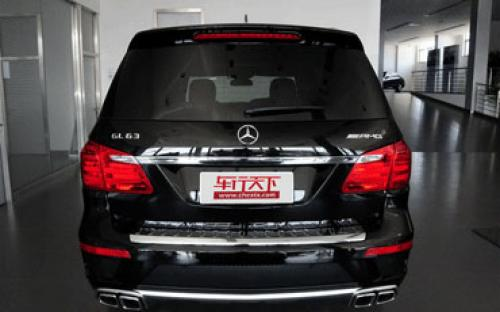### 奔驰AMG 奔驰GL级AMG14 种颜色可选2014款最低售价：198.00 万元起

5146(mm)1982(mm)1850(mm)##### 配置亮点：
• 胎压监测装置

• ISOFIX儿童座椅接口

• 车身稳定控制(ESC/ESP/DSC等)

• 电动天窗

• 定速巡航

• 后倒车雷达

• 真皮座椅

• GPS导航系统

• 氙气大灯

• 后视镜加热

• 提交

奔驰AMG 奔驰GL级AMG 绕车实拍• 奔驰AMG 奔驰GL级AMG 在售车型

排量 车型 厂商指导价 本地最低报价 购车工具
5.5L
GL63 AMG 7挡手自一体
198.00万
198.00万

奔驰AMG 奔驰GL级AMG 经销商

查看更多 >>

### 奔驰AMG 奔驰GL级AMG 动力加速

奔驰GL级AMG 0-100公里加速时间分布在 4.9-4.9秒 属于 高性能级

动力级别 加速时间 车型
高性能级(1款)
4.9s
GL63 AMG

奔驰AMG 奔驰GL级AMG 视频

奔驰AMG 奔驰GL级AMG 新闻资讯

# 实拍奔驰GL63 AMG 实力派的超级大胖子

评测 超过5684次关注

通常我总喜欢把全尺寸SUV称为胖子，因为它们往往尺寸夸张、行动迟缓，庞大的身躯经常会遭遇各种尴尬，比如调头堵了路，或是直接被停车场的限高吓丢了魂。不过AMG的...

# 成都车展 奔驰GL63 AMG独家解析

导购 超过4841次关注

作为奔驰家族最大的车型，GL在喜爱大车的中国和北美市场表现还不错，而AMG作为奔驰的高性能车型则一直受到性能车迷的追捧，那么当这两个元素合二为一，也就是我们今...

# 奔驰2014款GL63 AMG上市 售198.0万元

新闻 超过2753次关注

日前，我们了解到，奔驰2014款GL63AMG已经正式上市，目前该车的官方售价为198.0万元，其相比老款车型降低16.8万元。据悉，2014款GL63AMG将增加两项新的选装配置可选。

# 上海车展：奔驰GL63 AMG售214.8万

新闻 超过3368次关注

在本届上海车展上，奔驰发布了GL63AMG车型，售价为214.8万。该车装备5.5升V8双涡轮增压发动机，0-100km/h加速仅为4.9秒。

# 奔驰新款GL63 AMG车型上市 价格下调16万

新闻 超过3080次关注

近日，易车网获悉奔驰2014款GL63AMG车型上市的消息，新车相比上一代车型在价格上降低了16.8万元，目前官方售价为198万元。

# 奔驰AMG GT将10月首发 明年3月份销售

进口新车 超过5309次关注

近日AMG官方宣布，奔驰全新高性能跑车AMGGT将会在10月开幕的巴黎车展首发，随后于2015年3月份开始销售，而在2014年9月份会提前透露这款新产品的官方图片。

# 奔驰AMG动力计划曝光 布局2.0T发动机

进口新车 超过2948次关注

随着奔驰A45AMG和CLA45AMG等车型的亮相，拉开了奔驰AMG正式开始启用四缸涡轮增压发动机的序幕。日前，国外媒体报道，AMG未来将会更多的使用四缸涡轮增压发动机。

猜你喜欢

﻿
• 快速找车
• 选择品牌
• 选择品牌
• A  奥迪
• A  阿斯顿·马丁
• A  阿尔法·罗密欧
• B  宝沃
• B  布加迪
• B  巴博斯
• B  保时捷
• B  宾利
• B  奔驰
• B  宝马
• B  本田
• B  别克
• B  标致
• B  比亚迪
• B  宝骏
• B  北汽制造
• B  北汽新能源
• B  北汽幻速
• B  北汽威旺
• B  北京汽车
• B  奔腾
• B  北汽绅宝
• C  长安
• C  长安商用
• C  长城
• C  昌河
• D  大众
• D  道奇
• D  DS
• D  东南
• D  东风风神
• D  东风风行
• D  东风小康
• D  东风风度
• D  东风
• F  福特
• F  丰田
• F  菲亚特
• F  法拉利
• F  福田
• F  福迪
• F  福汽启腾
• G  观致
• G  广汽传祺
• G  广汽吉奥
• G  GMC
• H  红旗
• H  汉腾汽车
• H  哈弗
• H  哈飞
• H  海格
• H  海马
• H  华颂
• H  黄海
• H  华泰
• H  恒天
• J  吉利汽车
• J  捷豹
• J  Jeep
• J  江淮
• J  江铃
• J  金杯
• J  九龙
• J  金旅
• K  凯翼
• K  凯迪拉克
• K  克莱斯勒
• K  科尼塞克
• K  卡威
• K  开瑞
• L  路虎
• L  林肯
• L  劳斯莱斯
• L  兰博基尼
• L  雷克萨斯
• L  铃木
• L  雷诺
• L  理念
• L  力帆
• L  莲花汽车
• L  猎豹
• L  路特斯
• L  陆风
• M  马自达
• M  MG
• M  MINI
• M  玛莎拉蒂
• M  摩根
• M  迈凯轮
• N  纳智捷
• O  欧宝
• O  讴歌
• O  欧朗
• Q  奇瑞
• Q  起亚
• Q  启辰
• R  日产
• R  荣威
• R  瑞麒
• S  三菱
• S  斯威汽车
• S  萨博
• S  smart
• S  斯柯达
• S  斯巴鲁
• S  思铭
• S  双龙
• S  上汽大通
• S  双环
• T  特斯拉
• T  腾势
• W  沃尔沃
• W  五菱汽车
• W  五十铃
• W  威兹曼
• W  威麟
• X  现代
• X  雪佛兰
• X  雪铁龙
• X  西雅特
• Y  一汽
• Y  英菲尼迪
• Y  英致
• Y  依维柯
• Y  野马汽车
• Y  永源
• Z  众泰
• Z  中华
• Z  中兴
• Z  知豆
• 选择车系
• 选择车系
• 车型对比
• 选择品牌
• 选择品牌
• A  奥迪
• A  阿斯顿·马丁
• A  阿尔法·罗密欧
• B  宝沃
• B  布加迪
• B  巴博斯
• B  保时捷
• B  宾利
• B  奔驰
• B  宝马
• B  本田
• B  别克
• B  标致
• B  比亚迪
• B  宝骏
• B  北汽制造
• B  北汽新能源
• B  北汽幻速
• B  北汽威旺
• B  北京汽车
• B  奔腾
• B  北汽绅宝
• C  长安
• C  长安商用
• C  长城
• C  昌河
• D  大众
• D  道奇
• D  DS
• D  东南
• D  东风风神
• D  东风风行
• D  东风小康
• D  东风风度
• D  东风
• F  福特
• F  丰田
• F  菲亚特
• F  法拉利
• F  福田
• F  福迪
• F  福汽启腾
• G  观致
• G  广汽传祺
• G  广汽吉奥
• G  GMC
• H  红旗
• H  汉腾汽车
• H  哈弗
• H  哈飞
• H  海格
• H  海马
• H  华颂
• H  黄海
• H  华泰
• H  恒天
• J  吉利汽车
• J  捷豹
• J  Jeep
• J  江淮
• J  江铃
• J  金杯
• J  九龙
• J  金旅
• K  凯翼
• K  凯迪拉克
• K  克莱斯勒
• K  科尼塞克
• K  卡威
• K  开瑞
• L  路虎
• L  林肯
• L  劳斯莱斯
• L  兰博基尼
• L  雷克萨斯
• L  铃木
• L  雷诺
• L  理念
• L  力帆
• L  莲花汽车
• L  猎豹
• L  路特斯
• L  陆风
• M  马自达
• M  MG
• M  MINI
• M  玛莎拉蒂
• M  摩根
• M  迈凯轮
• N  纳智捷
• O  欧宝
• O  讴歌
• O  欧朗
• Q  奇瑞
• Q  起亚
• Q  启辰
• R  日产
• R  荣威
• R  瑞麒
• S  三菱
• S  斯威汽车
• S  萨博
• S  smart
• S  斯柯达
• S  斯巴鲁
• S  思铭
• S  双龙
• S  上汽大通
• S  双环
• T  特斯拉
• T  腾势
• W  沃尔沃
• W  五菱汽车
• W  五十铃
• W  威兹曼
• W  威麟
• X  现代
• X  雪佛兰
• X  雪铁龙
• X  西雅特
• Y  一汽
• Y  英菲尼迪
• Y  英致
• Y  依维柯
• Y  野马汽车
• Y  永源
• Z  众泰
• Z  中华
• Z  中兴
• Z  知豆
• 选择车系
• 选择车系
• 选择车型
• 选择车型
• 意见反馈